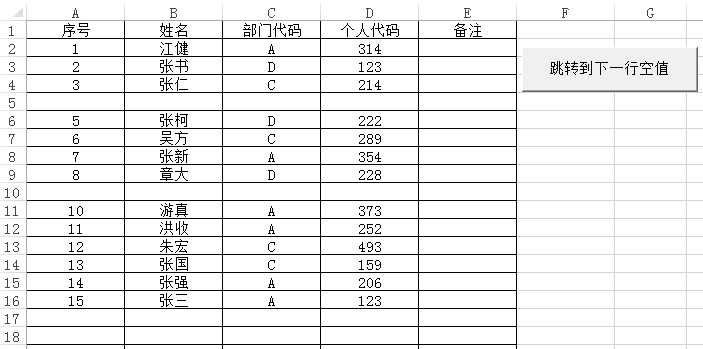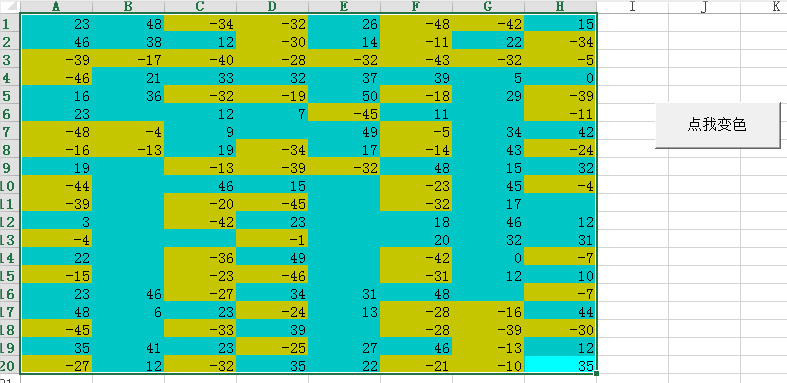• 如果我们选中了单元格里的部分内容，想在选中内容的前后分别插入不同的字符，这个用VBA怎么做呢？这个直接在Excel单元格中操作是没有办法实现的，或者说实现难度非常之大。我们可以换个思路，把单元格内容体现在...我们都知道Excel单元格中可以存储各种文字内容。

如果我们选中了单元格里的部分内容，想在选中内容的前后分别插入不同的字符，这个用VBA怎么做呢？

这个直接在Excel单元格中操作是没有办法实现的，或者说实现难度非常之大。

我们可以换个思路，把单元格的内容体现在窗体文本框中，通过文本框来选择要操作的部分内容。

如下图所示在文本框中选择要添加的部分内容，然后选择要添加的XML标签，单击”开始添加”即可在选中的文本内容前后添加要添加的文本内容，最后将添加后的文本返回到单元格中。

这里需要了解的是在文本框控件中，可以使用SelStart属性返回选中的文本内容的起始位置，SelLength属性返回选中的文本内容的字符长度，SelText 属性返回选中的文本内容。

核心代码如下：

Visual Basic

Private Sub CmdOK_Click()

With UserForm1

sText = .TextBox1.Text

sTag = .ComboBox1.Text

If Len(sText) > 0 Then

iStart = .TextBox1.SelStart

If iStart = 0 Then iStart = 1

sSText = .TextBox1.SelText

sNText = "" & sSText & "" & sTag & ">"

sText = Left(sText, iStart - 1) & VBA.Replace(sText, sSText, sNText, iStart, 1)

.TextBox1.Text = sText

Excel.ActiveCell = sText

End If

End With

End Sub

1

2

3

4

5

6

7

8

9

10

11

12

13

14

15

PrivateSubCmdOK_Click()

WithUserForm1

sText=.TextBox1.Text

sTag=.ComboBox1.Text

IfLen(sText)>0Then

iStart=.TextBox1.SelStart

IfiStart=0TheniStart=1

sSText=.TextBox1.SelText

sNText=""&sSText&""&sTag&">"

sText=Left(sText,iStart-1)&VBA.Replace(sText,sSText,sNText,iStart,1)

.TextBox1.Text=sText

Excel.ActiveCell=sText

EndIf

EndWith

EndSub

通过上述代码，就可以随心所意的选择要修改的部分文本内容，然后在其前后添加文本内容。

怎么样？今天介绍的思路你学会了吗？展开全文• 运行后就会弹出个警告框，提示用户输入内容，输入后就会在A1单元格显示了，但是也存在一个问题，就是取消后呢，原来在A1单元格内容就会被清空。 所以改良一下，也把“输入数字这个功能再完善一下”。 Sub ...

继续讲讲单元格的操作

一、提示用户输入值

Sub SetValue()
Sheets("sheet3").Range("a1") = InputBox("输入一个数字")
End Sub

运行后就会弹出个警告框，提示用户输入内容，输入后就会在A1单元格显示了，但是也存在一个问题，就是取消后呢，原来在A1单元格的内容就会被清空。

所以改良一下，也把“输入数字这个功能再完善一下”。

Sub SetValue()
Dim myInput As Variant
myInput = InputBox("输入一个数字")
If myInput <> "" Then
If IsNumeric(myInput) Then
Sheets("sheet3").Range("a1") = myInput
Else
MsgBox "输入的不是数字"
End If
Else
MsgBox "输入的内容为空值"
End If
End Sub

在判断是否是数字上，如果用WorksheetFunction.IsNumber判断可能会出错。用户在输入123后，WorksheetFunction.IsNumber仍然会识别成文本格式。经过资料查询，解释是这样的：

WorksheetFunction.IsNumber：函数中的数值参数是无法转换的。例如，大部分其他函式中其中一个数字是必要，文字值19会转换的数字19。不过，在公式中ISNUMBER（“19”）、19不会转换文字值，且IsNumber函数会传回False。

IsNumeric：如果在整个表达式被视为一个数字就为真，否则，它会传回False。如果_表达式_为日期表达式IsNumeric会传回False。

所以咱们就知道为什么IsNumeric用来判断用户输入是否数字可以，而WorksheetFunction.IsNumber不行啦。

二、在空值输入内容

在空值输入内容的第一步当然是确定空值的位置啦，运行后跳转到空值的地方进行输入。我这里主要用到昨天的Range.End(xldown)来确定下一行的空值。

Sub SetBlankValue()
Dim rowNum As Integer
rowNum = Range("a1").End(xlDown).Row + 1
Range("a" & rowNum).Select
End Sub是不是很简单啦。

三、计算选中单元格 数量

MsgBox Selection.Count

框柱单元格后运行就出来了，一句话完事儿~

如果要有选择多个选区，想知道多个选取的数量，用以下方式

MsgBox Selection.Areas.Count

四、有效遍历选区

有效遍历选区一般是对选区内有值的进行遍历操作，不然大量空值存在就很浪费时间，下面是对用户框住的内容进行判断，小于0的就成黄色，大于等于0的就蓝色。

Sub BianLi()
Dim cell As Range
If TypeName(Selection) <> "Range" Then Exit Sub
Application.ScreenUpdating = False
For Each cell In Selection
If cell.Value < 0 Then
cell.Interior.Color = RGB(255, 255, 0)
Else
cell.Interior.Color = RGB(0, 255, 255)
End If
Next
End Sub框选后确实变色了，但是呢如果选中整行整列的话，会比较慢，工作表有个叫UsedRange的方法，官方解释【返回一个**Range** 对象, 该对象代表指定工作表上的所用区域。】也就是咱们用了多少单元格意思。输出个已使用单元格数量试试

MsgBox (Sheets(1).UsedRange.Count)

然后我们就可以根据用户框住的区域和这个UsedRange区域进行交叉匹配，匹配到了的说明有值，需要进行运算

Sub BianLi2()
Dim cell As Range, rng As Range
If TypeName(Selection) <> "Range" Then Exit Sub
Application.ScreenUpdating = False
Set rng = Application.Intersect(Selection, ActiveSheet.UsedRange)
For Each cell In rng
If cell.Value < 0 Then
cell.Interior.Color = RGB(255, 255, 0)
Else
cell.Interior.Color = RGB(0, 255, 255)
End If
Next
End Sub

结果和上图相似，但是速度会稍稍快了点，但是也带来一个问题，如果用户点了空白区域，既没有内容，也没有使用，所以可能会报错哟，所以还需要改进一下。

此刻需要用表达式。Range.specialcells(Type, Value)来区分一下，该函数返回一个Range对象, 该对象代表与指定类型和值匹配的所有单元格。第一个参数是要包含的单元格，必填；第二个参数是可选的，写上要返回哪些类型的单元格

所以咱们把有值的Range用这个函数拆成2部分，数字的，就做判断，不是数字的，就不管了

Sub BianLi3()
Dim numCells As Range, cell As Range
If TypeName(Selection) <> "Range" Then Exit Sub
Application.ScreenUpdating = False

'xlConstants 意思是单元格是值，xlNumbers意思就是数字
Set numCells = Selection.SpecialCells(xlConstants, xlNumbers)

For Each cell In numCells
If cell.Value < 0 Then
cell.Interior.Color = RGB(255, 255, 0)
Else
cell.Interior.Color = RGB(0, 255, 255)
End If
Next
End Sub

展开全文• 如何判断当前单元格在窗口中只露出一半？ 比如下面的three，它只露出一半，此时你点击它，窗口也不会自动帮你往下移动滚轮以确保完全看到这一个单元格...小程序 java c++ 开发语言
• 判断j列数据>m列数据>l列数据>m列数据，否则提示错误 Sub test() n = 5 '表示从第5行开始 While (n <= 147) '到147行终止 c1 = Range("j" + CStr(n)).Value 'j列判断 c2 = Range("k" + CStr(n))....

判断j列数据>m列数据>l列数据>m列数据，否则提示错误

Sub test()
n = 5           '表示从第5行开始
While (n <= 147)        '到147行终止
c1 = Range("j" + CStr(n)).Value         'j列判断
c2 = Range("k" + CStr(n)).Value
c3 = Range("l" + CStr(n)).Value
c4 = Range("m" + CStr(n)).Value
If c1 < c2 Or c1 < c3 Or c1 < c4 Or c2 < c3 Or c2 < c4 Or c3 < c4 Then      '关键判断步骤
MsgBox "第" + CStr(n) + "个单元格不符合逻辑", vbOKCancel, "错误"
End If
n = n + 1
Wend
MsgBox "成功", vbOKCancel, "成功"
End Sub

展开全文vba
• Function isNum(rng) As Boolean Dim i As Integer On Error Resume Next i = rng isNum = Not Err.Number = 13 End Function Sub sdk() For Each rng In Range("U2:Z527") If isNum(rng) = False Then rng....

Function isNum(rng) As Boolean
Dim i As Integer
On Error Resume Next
i = rng
isNum = Not Err.Number = 13
End Function

Sub sdk()
For Each rng In Range("U2:Z527")
If isNum(rng) = False Then rng.Select
Next
End Sub

展开全文vba
• 前言：最近在做一个地址标准化的竞赛，里面包括了地址提取、分词、清洗、匹配等一...如上图所示，我想要的结果就是根据C列，判断B列里是否有一样的内容，如果有的话就用红色标记，使用VBA很简单，不过也查了蛮多资料vba
• 我们经常需要监督Excel工作表中单元格的值是否变化，例如我们客服有一个报表要通报，报表的内容来自很多地方，需要将这些数据复制到这个表中，由于数据较多，为了防止有遗漏，希望更新后的数据有个标志，这样没有...
• 我们一般触发或者执行一段宏命令都是通过自定义按钮或者自定义快速访问栏调用相关宏命令，前一段时间在CSDN中回答网友提问的问题时，我无意中测试成功了一些Excel的内置事件，其中符合网友要求的就是单元格变更事件...excel vba
• A中的第4列是否在B中的第9列包含 Sub 宏1() m = Sheets("B").UsedRange.Rows.Count For i = 3 To 3 'Sheets("A").UsedRange.Rows.Count s = Sheets("A").Cells(i, 4) Sheets("A").Cells(i, 5) = "" ...VBA
• 你的VBA过程可能需要查明某个具体单元格的格式。我们可以使用NumberFormat属性来找回单元格格式： ?Range("A1").NumberFormat 在立即窗口输入上面的问题后，Excel显示“General” Range("A1").NumberFormat =...excel vba 程序设计
• 检查 单元格中文 Sub test() For i = 2 To 358010 If (Len(Cells(i, 2)) < LenB(StrConv(Cells(i, 2), vbFromUnicode))) Then Cells(i, 3) = 1 End If Next i End Sub 检查单元格字符串 Sub test() For i...
• 系列文章目录 提示：这里可以添加系列文章...例如：随着人工智能的不断发展，机器学习这门技术也越来越重要，很多人都开启了学习机器学习，本文就介绍了机器学习的基础内容。 提示：以下是本篇文章正文内容，下面案例vba excel
• Debug.Print "条目" & k & "：" & Rng.Value & ActiveSheet.Name '输出当前工作表内容 last_row = ThisWorkbook.Sheets("进出库").Cells(Rows.Count, 1).End(xlUp).Row '最后一行位置 ' n = n + 1 '判断行数 ...python 爬虫 pycharm
• 使用vba在word中处理表格时,有时要判断这一行表格是否为空白,无内容,以便中止或者跳过读取数据,编辑内容。 可以使用Table对象的Rows属性返回具体的行，然后判断第一个单元格内容.再做下一步操作. 在word中,空白...
• 对于合并单元格这里提供”从上到下“和“从下到上”合并单元格两种方式，”从上到下“的方法需要记录当前列有多少个相同的单元格判断相应的单元格是否是合并单元格，过程相对来说繁琐一些，“从下到上”的方法则...
• Excel VBA：单元格错误值

千次阅读 2017-01-05 21:27:48
可将单元格错误值插入单元格，或者用 CVErr函数测试单元格的值是否为某个错误值。单元格错误值可为以下 XlCVError 常量之一。 常量 错误号 单元格错误值 xlErrDiv0 2007 #DIV/0! xlErrNA 2042...
• Sub lsy() Range("f9:f10").Font.ColorIndex = 2 Range("g9").Font.ColorIndex = 2 If Range("g10") Range("g10").Font.ColorIndex = 3 Else Range("g10").Font.ColorIndex = 2vba excel
• 最近因某项目的客户需求，需要使用vba，监听某工作簿的某工作表内单元格的值是否发生变化（在已有值上手动修改或者在空白处添加新值），如果有变化设置变化后的单元格的字体为红色。 作为初学vba的小白，在网上查了...vba
• Excel：VBA 数据分析 写一个for循环的单元格判断程序（四 还是那张测试表。 本文要实现的逻辑是：在 F列 实现对 E列 的判断并显示结果。当E列 的单元格 分数 大于 90 分时，则 F列 应该显示判断结果为优秀；当 E列 ...excel vba 数据分析
• Excel VBA Range单元格操作实例

千次阅读 2020-02-16 21:51:53
4.2取得最后一个非空单元格 xlDown/xlToRight/xlToLeft/xlUp Dim ERow as Long Erow=Range("A" & Rows.Count).End(xlUp).Row 4.3 复制单元格区域 注意：使用PasteSpecial方法时指定xlPasteAll（粘贴全部），并...excel vba
• 有些部门发来的表格完全是按照以前的...所以为了准确获取表格所在位置，只根据有无内容判断表格的行号，我写了这样一段程序 Sub 判断当前表格的数据区域() Dim datarange As Range, rangerow, rangecol, maxrow...后端 vba
• VBA示例之 在特定的区域内查找文本,返回值是包含查找文本的单元格，供初学者参考，大牛勿进~~~~~~~
• 众所周知EXCEL是办公利器，特别是在报表处理工作较多的办公环境下，一个简单的EXCEL VBA的小技能就可以剩下你大量的时间。 这不是重点，最最重点的是，这个小小的效率提升会减少工作里重复劳动的烦躁感，幸福度upup...
• 判断Excel单元格是否处于编辑状态 以下是平时收集到的一个判断表格单元格是否处理编辑状态的代码，记不住是从哪弄的，转给大家，供学习参考。 '//取得类名 Private Declare Function GetClassName Lib "user32" ...vba excel wps
• VBA单元格背景色类型

千次阅读 2019-12-30 16:17:58VBA
• [a5] = ActiveCell.Cells '获取选中单元格内容 [a6] = Target.Address(0, 0) '获取选中单元格名称（字符名称 例如 C5 ) [a7] = ActiveCell.Cells.Row '获取选中单元格的行编号 数值 [a8] = ActiveCell.Cells....vba获取选中单元格
• excel基本操作，创建，读写，公式设置，判断是否为合并单元格
• 单击单元格进入编辑状态 Private Sub Worksheet_SelectionChange(ByVal Target As Range) '实现单击单元格进入编辑状态 If Target.Column = 3 And Target.Count = 1 Then '条件为第三列并且只选中一个单元格时 If ...
• 意思就是当前应用程序(excel)下面的已经打开的第一个工作薄下面的第一个工作表里面的A1单元格，但一般情况下我们不需要这么麻烦，如果我们只对当前的表格操作，前面的都可以省略，只需要写range(“A1
• 1.EXCEL VBA遍布文件夹的操作 关于VBA遍历文件夹主要用的是提供的Application.FileDialo函数来由个人进行自由选择，通过获取选择的文件夹地址之后，通过Dir函数来匹配选取文件夹下的相应的文档。相应的VBA程序代码......